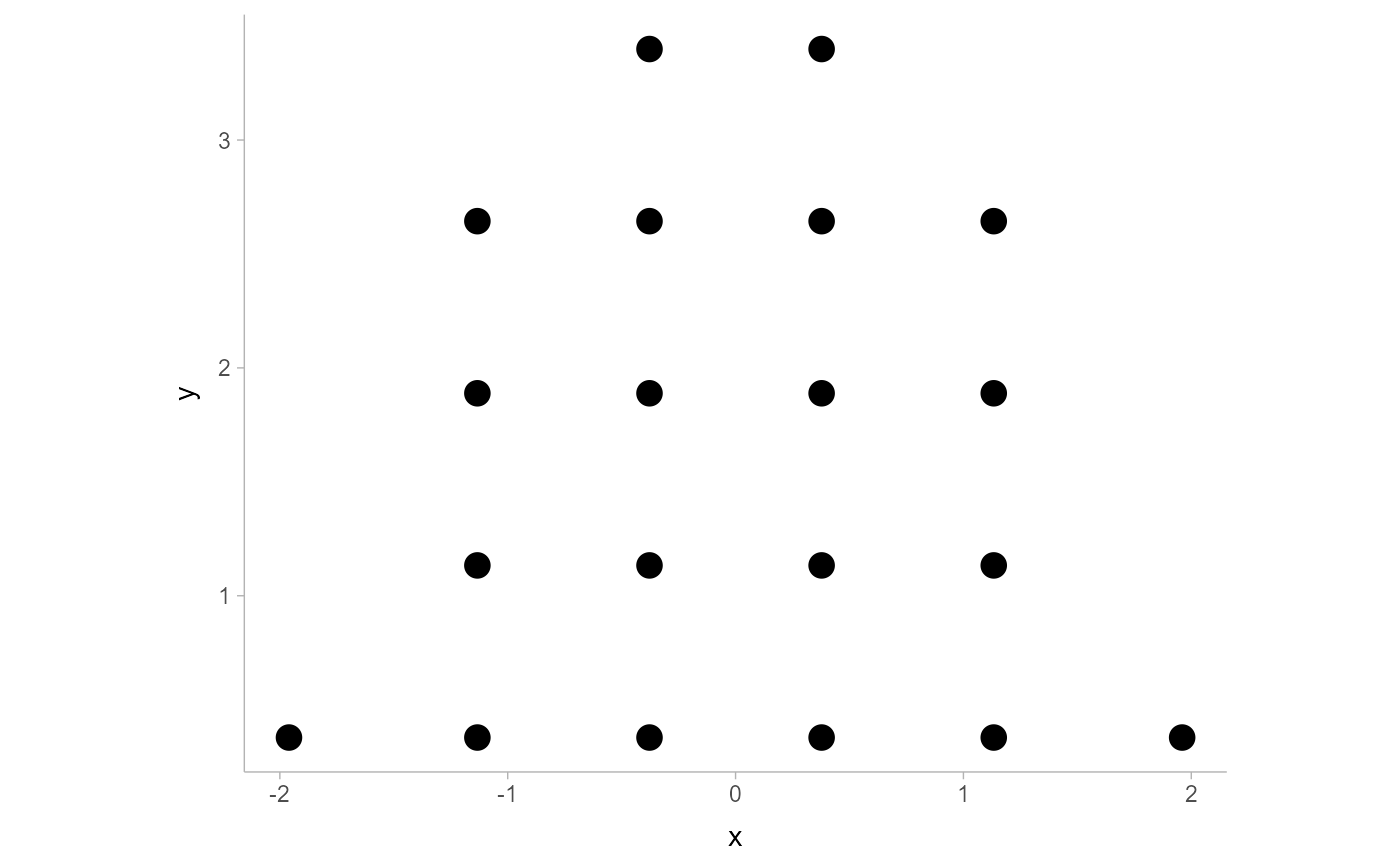Searches for a nice-looking bin width to use to draw a dotplot such that the height of the dotplot fits within a given space (maxheight).

find_dotplot_binwidth(
x,
maxheight,
heightratio = 1,
stackratio = 1,
layout = c("bin", "weave", "hex", "swarm", "bar")
)

## Arguments

x

numeric vector of values

maxheight

maximum height of the dotplot

heightratio

ratio of bin width to dot height

stackratio

ratio of dot height to vertical distance between dot centers

layout

The layout method used for the dots:

• "bin" (default): places dots on the off-axis at the midpoint of their bins as in the classic Wilkinson dotplot. This maintains the alignment of rows and columns in the dotplot. This layout is slightly different from the classic Wilkinson algorithm in that: (1) it nudges bins slightly to avoid overlapping bins and (2) if the input data are symmetrical it will return a symmetrical layout.

• "weave": uses the same basic binning approach of "bin", but places dots in the off-axis at their actual positions (unless overlaps = "nudge", in which case overlaps may be nudged out of the way). This maintains the alignment of rows but does not align dots within columns.

• "hex": uses the same basic binning approach of "bin", but alternates placing dots + binwidth/4 or - binwidth/4 in the off-axis from the bin center. This allows hexagonal packing by setting a stackratio less than 1 (something like 0.9 tends to work).

• "swarm": uses the "compactswarm" layout from beeswarm::beeswarm(). Does not maintain alignment of rows or columns, but can be more compact and neat looking, especially for sample data (as opposed to quantile dotplots of theoretical distributions, which may look better with "bin", "weave", or "hex").

• "bar": for discrete distributions, lays out duplicate values in rectangular bars.

## Value

A suitable bin width such that a dotplot created with this bin width and heightratio should have its tallest bin be less than or equal to maxheight.

## Details

This dynamic bin selection algorithm uses a binary search over the number of bins to find a bin width such that if the input data (x) is binned using a Wilkinson-style dotplot algorithm the height of the tallest bin will be less than maxheight.

This algorithm is used by geom_dotsinterval() (and its variants) to automatically select bin widths. Unless you are manually implementing you own dotplot grob or geom, you probably do not need to use this function directly

bin_dots() for an algorithm can bin dots using bin widths selected by this function; geom_dotsinterval() for geometries that use these algorithms to create dotplots.

## Examples


library(dplyr)
library(ggplot2)

x = qnorm(ppoints(20))
binwidth = find_dotplot_binwidth(x, maxheight = 4, heightratio = 1)
binwidth
#>  0.755415

bin_df = bin_dots(x = x, y = 0, binwidth = binwidth, heightratio = 1)
bin_df
#> # A tibble: 20 × 3
#>         x     y   bin
#>     <dbl> <dbl> <dbl>
#>  1 -1.96  0.378     1
#>  2 -1.13  0.378     2
#>  3 -1.13  1.13      2
#>  4 -1.13  1.89      2
#>  5 -1.13  2.64      2
#>  6 -0.378 0.378     3
#>  7 -0.378 1.13      3
#>  8 -0.378 1.89      3
#>  9 -0.378 2.64      3
#> 10 -0.378 3.40      3
#> 11  0.378 0.378     4
#> 12  0.378 1.13      4
#> 13  0.378 1.89      4
#> 14  0.378 2.64      4
#> 15  0.378 3.40      4
#> 16  1.13  0.378     5
#> 17  1.13  1.13      5
#> 18  1.13  1.89      5
#> 19  1.13  2.64      5
#> 20  1.96  0.378     6

# we can manually plot the binning above, though this is only recommended
# if you are using find_dotplot_binwidth() and bin_dots() to build your own
# grob. For practical use it is much easier to use geom_dots(), which will
# automatically select good bin widths for you (and which uses
# find_dotplot_binwidth() and bin_dots() internally)
bin_df %>%
ggplot(aes(x = x, y = y)) +
geom_point(size = 4) +
coord_fixed()